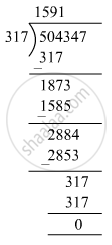# The product of two numbers is 504347. If one of the numbers is 317, find the other. - Mathematics

Fill in the Blanks

The product of two numbers is 504347. If one of the numbers is 317, find the other.

#### SolutionLet x and y be the two numbers.
Product of the two numbers = x × y = 504347
If x = 317, we have:
317 × y = 504347
⇒ y = 504347 ÷ 317
y= 1591
∴ The other number is 1591.

Concept: Concept for Whole Numbers
Is there an error in this question or solution?

#### APPEARS IN

RS Aggarwal Class 6 Mathematics
Chapter 3 Whole Numbers
Exercise 3E | Q 5 | Page 56